# Circle chord

What is the length d of the chord circle of diameter 50 dm, if the distance from the center circle is 21 dm?

Result

x =  54.259 dm

#### Solution:Leave us a comment of this math problem and its solution (i.e. if it is still somewhat unclear...):Be the first to comment!#### Following knowledge from mathematics are needed to solve this word math problem:

Pythagorean theorem is the base for the right triangle calculator. See also our trigonometric triangle calculator.

## Next similar math problems:

1. The chordSide of the triangle inscribed in a circle is a chord passing through circle center. What size are the internal angles of a triangle, if one of them is 40°?
2. Two chordsCalculate the length of chord AB and perpendicular chord BC to circle if AB is 4 cm from the center of the circle and BC 8 cm from the center of the circle.
3. Semicircle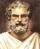In the semicircle with center S and the diameter AB is constructed equilateral triangle SBC. What is the magnitude of the angle ∠SAC?
4. RectangleIn rectangle with sides, 6 and 3 mark the diagonal. What is the probability that a randomly selected point within the rectangle is closer to the diagonal than to any side of the rectangle?
5. Hypotenuse - RTA triangle has a hypotenuse of 55 and an altitude to the hypotenuse of 33. What is the area of the triangle?
6. Complete constructionConstruct triangle ABC if hypotenuse c = 7 cm and angle ABC = 30 degrees. / Use Thales' theorem - circle /. Measure and write down the length of legs.
7. Inscribed triangle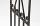To a circle is inscribed triangle so that the it's vertexes divide circle into 3 arcs. The length of the arcs are in the ratio 2:3:7. Determine the interior angles of a triangle.
8. Coal mine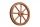The towing wheel has a diameter of 1.7 meters. How many meters does the elevator cage lower when the wheel turns 32 times?
9. Circle tangentIt is given to a circle with the center S and radius 3.5 cm. Distance from the center to line p is 6 cm. Construct a circle tangent n which is perpendicular to the line p.
10. Circle describedThe radius of the circle described to the right triangle with 6 cm long leg is 5 cm. Calculate the circumference of this triangle.
11. The bridge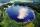Across the circle lakepasses through its center bridge over the lake. At three different locations on the lake shore are three fishermen A, B, C. Which of fishermen see the bridge under the largest angle?
12. Circumferential angleVertices of the triangle ΔABC lies on circle and divided it into arcs in the ratio 2:2:9. Determine the size of the angles of the triangle ΔABC.
13. OK circleCalculate the radius (circumradius) of the circle described right triangle with hypotenuse long 33 and one cathetus long 17.
14. Right triangleDraw a right triangle ABC if |AB| = 5 cm |BC| = 3 cm, |AC| = 4 cm. Draw Thales circle above the hypotenuse of the triangle ABC.
15. Tangents constructCircle is given k (S; 2.5 cm) and an outer line p. Construct a tangent t of the circle that has with a line p angle 60°. How many solutions has the task?
16. Same area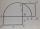There is a given triangle. Construct a square of the same area.
17. The cylinder 2Find the volume and the lateral area of a cylinder of height 12 inches and a base radius of 4 inches.